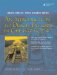# Class Definitions

C++ has another datatype called class that is very similar to struct. A simple class definition looks like this:

```class className {
members
};
```

The first line of the class definition is called the classHead. The features of a class include data members, member functions, and access specifiers. Member functions are used to manipulate or manage the data members.

After a class has been defined, the class name can be used as a type for variables, parameters, and returns from functions. Variables of a class type are called objects, or instances, of a class.

Member functions for class T specify the behavior of all objects of type T and have access to all members of the class. Non-member functions normally manipulate objects indirectly by calling member functions. The set of values of the data members of an object is called the state of the object.

To define a class (or any other type) we generally place the definition in a header file, preferably with the same name as the class and with the .h extension. Example 2.3 shows a class definition defined in a header file.

Example 2.3. src/classes/fraction.h

 ```#ifndef _FRACTION_H_ #define _FRACTION_H_ #include using namespace std; class Fraction { public: void set(int numerator, int denominator); double toDouble() const; string toString() const; private: int m_Numerator; int m_Denominator; }; #endif ```

Header files are #included in other files by the preprocessor. To prevent a header file from accidentally being #included more than once in any compiled file, we wrap it with #ifndef-#define . . . #endif preprocessor macros (see Section C.1).

Generally, we place the definitions of member functions outside the class definition in a separate implementation file with the .cpp extension.

The definition of any class member outside the class definition requires a scope resolution operator of the form ClassName:: before its name. The scope resolution operator tells the compiler that the scope of the class extends beyond the class definition and includes the code between the :: and the closing brace of the function definition.

Example 2.4 is an implementation file that corresponds to Example 2.3.

Example 2.4. src/classes/fraction.cpp

 ```#include "fraction.h" #include void Fraction::set(int nn, int nd) { m_Numerator = nn; m_Denominator = nd; } double Fraction::toDouble() const { return 1.0 * m_Numerator / m_Denominator; } string Fraction::toString() const { ostringstream sstr; <-- 1 sstr << m_Numerator << "/" << m_Denominator; return sstr.str(); <-- 2 } ```   (1)a stream we write to, but that does not get output anywhere (2)convert the stream just written to a string

Every identifier has a scope (see Section 20.2), a region of code where a name is "known" and accessible. Class member names have class scope. Class scope includes the entire class definition, regardless of where the identifier was declared, as well as inside each member function definition, starting at the :: and ending at the closing brace of the definition.

So, for example, the members, Fraction::m_Numerator and Fraction::m_Denominator are visible inside the definitions of Fraction::toString() and Fraction::toDouble() even though those function definitions are in a separate file.

### Member Access SpecifiersAn Introduction to Design Patterns in C++ with Qt 4
ISBN: 0131879057
EAN: 2147483647
Year: 2004
Pages: 268